Next: Spatial Up: Continuous Weights Mixture Model Previous: Non-spatial without Class Proportions

### Non-spatial with Class Proportions

We can approximate the distribution in equation 5 by replacing the discrete labels,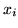, with continuous weights vectors,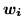: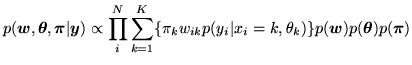(13)

where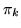are the global class proportion parameters defined in equation 4 and represent the proportion of each class in the mixture model, and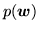is given by equations 10-12. Note that when we infer on the posterior,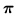will depend upon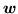in same way thatdepends on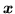in the discrete labels mixture model.